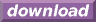#Calcute: freeware calculator

## Hyperbolic Cosine (cosh)

Calcute implements the hyperbolic cosine function with the cosh function.

```cosh(1)
1.54308063482
```

It is defined in terms of the exponential function as cosh(x) = (exp(x) + exp(-x)) / 2.

A similarity exists between the trigonometric cosine and the hyperbolic cosine functions. While the former is best described graphically using a unit circle with equation (x2 + y2) = 1, the hyperbolic cosine follows the unit hyperbola with equation (x2 - y2) = 1 instead.